# Strategies for Spatial Joins Recall Spatial Join Example

• Slides: 20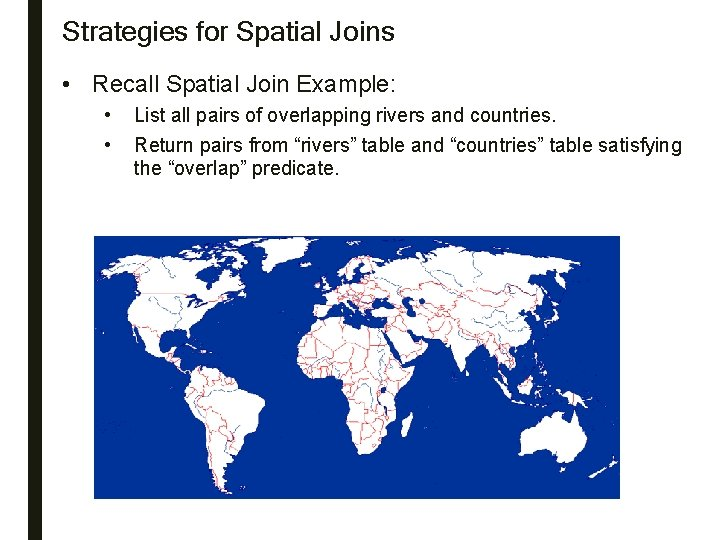Strategies for Spatial Joins • Recall Spatial Join Example: • • List all pairs of overlapping rivers and countries. Return pairs from “rivers” table and “countries” table satisfying the “overlap” predicate.Strategies for Spatial Joins - Continued • List of strategies • Nested loop: • • Test all possible pairs for spatial predicate All rivers are paired with all countries • Space Partitioning: • • Test pairs of objects from common spatial regions only Rivers in Africa are tested with countries in Africa only • Tree Matching • • Hierarchical pairing of object groups from each table Other, e. g. spatial-join-index based, external plane-sweep, …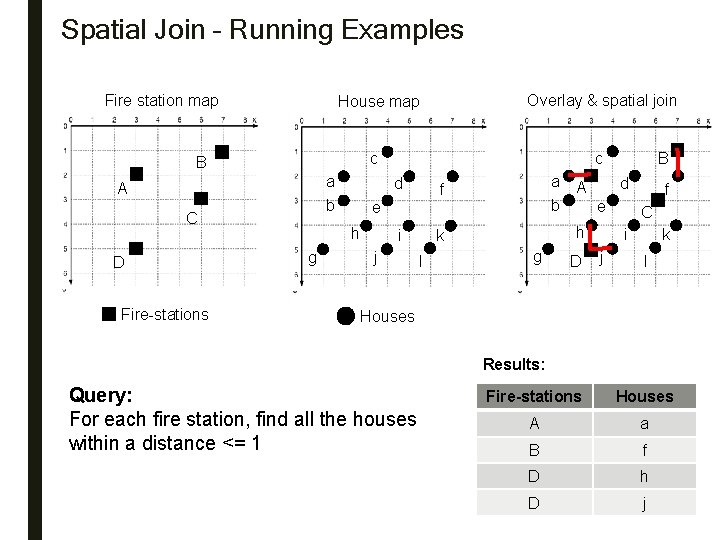Spatial Join – Running Examples Fire station map a b A C Fire-stations c c B D Overlay & spatial join House map d e h g i j a b f l d A e h k g B D f C i j k l Houses Results: Query: For each fire station, find all the houses within a distance <= 1 Fire-stations Houses A a B f D h D j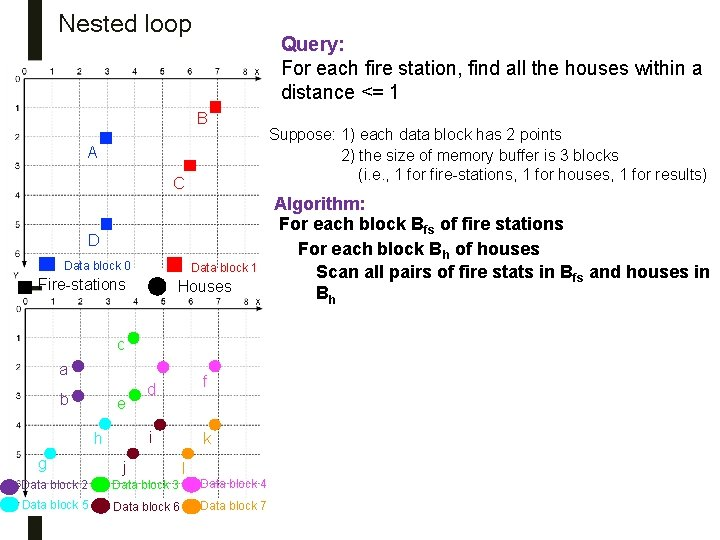Nested loop Query: For each fire station, find all the houses within a distance <= 1 B A C D Data block 0 Data block 1 Fire-stations Houses c a b e i h g Data block 2 3 block 5 Data f d j k l Data block 3 Data block 4 Data block 6 Data block 7 Suppose: 1) each data block has 2 points 2) the size of memory buffer is 3 blocks (i. e. , 1 for fire-stations, 1 for houses, 1 for results) Algorithm: For each block Bfs of fire stations For each block Bh of houses Scan all pairs of fire stats in Bfs and houses in BhNested loop B A C Query: For each fire station, find all the houses within a distance <= 1 Suppose: 1) each data block has 2 points 2) the size of memory buffer is 3 blocks (i. e. , 1 for fire-stations, 1 for houses, 1 for results) Algorithm: For each block Bfs of fire stations D Data block 0 Data block 1 Fire-stations Houses For Block 0, traverse through Blocks 2 -7 For Block 1, traverse through Blocks 2 -7 c a b e g Data block 2 3 block 5 Data f d j Cost: # blocks for fire stations * # blocks for houses = 2*6 = 12 Fire stations i h For each block Bh of houses Scan all pairs of fire stats in Bfs and houses in Bh k l Data block 3 Data block 4 Data block 6 Data block 7 HousesNested loop with Index for Inner Loop B A C Query: For each fire station, find all the houses within a distance <= 1 Suppose an R-tree (primary index) is available for the houses. D Data block 0 X Data block 2 3 Data block 5 d f i k Y e h Y g X c a b Data block 1 j l Data block 3 Data block 4 Data block 6 Data block 7 a b c e d f g h i j k lNested loop with Index for Inner Loop B A For each block of fire stations, create MOBR with length of 1. C D Data block 0 X b Data block 2 3 Data block 5 d f i k e h Y g Data block 1 c a j Query: For each fire station, find all the houses within a distance <= 1 l Data block 3 Data block 4 Data block 6 Data block 7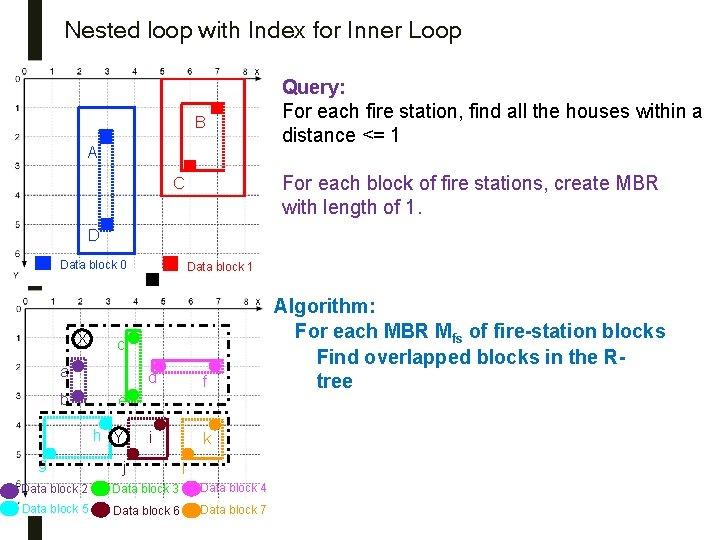Nested loop with Index for Inner Loop B A Query: For each fire station, find all the houses within a distance <= 1 For each block of fire stations, create MBR with length of 1. C D Data block 0 X c a b Data block 2 3 Data block 5 d f i k e h Y g Data block 1 j l Data block 3 Data block 4 Data block 6 Data block 7 Algorithm: For each MBR Mfs of fire-station blocks Find overlapped blocks in the Rtree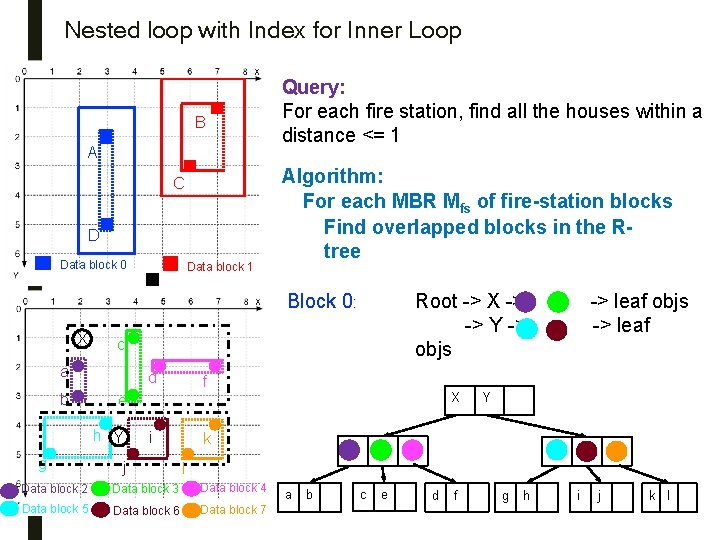Nested loop with Index for Inner Loop B A C D Data block 0 Data block 1 Query: For each fire station, find all the houses within a distance <= 1 Algorithm: For each MBR Mfs of fire-station blocks Find overlapped blocks in the Rtree Root -> X -> -> Y -> objs Block 0: X c a b d g Data block 2 3 Data block 5 X i j -> leaf objs -> leaf f e h Y , , Y k l Data block 3 Data block 4 Data block 6 Data block 7 a b c e d f g h i j k l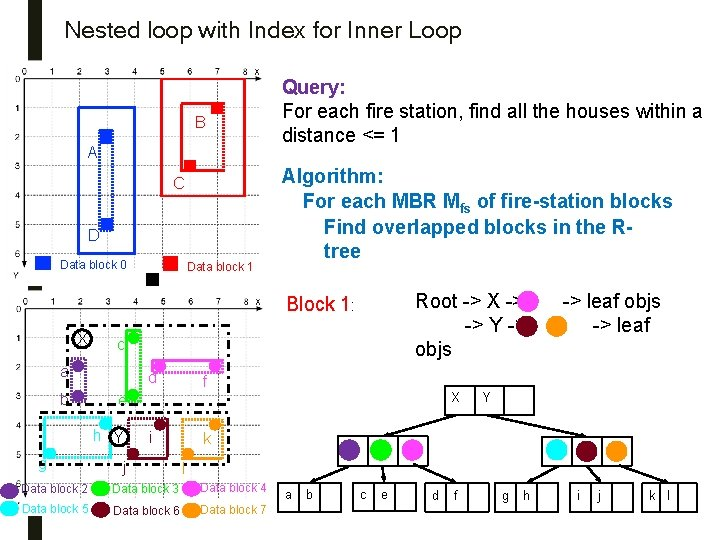Nested loop with Index for Inner Loop B A C D Data block 0 Data block 1 Query: For each fire station, find all the houses within a distance <= 1 Algorithm: For each MBR Mfs of fire-station blocks Find overlapped blocks in the Rtree Root -> X -> -> Y -> objs Block 1: X c a b d g Data block 2 3 Data block 5 f X e h Y i j -> leaf objs , -> leaf Y k l Data block 3 Data block 4 Data block 6 Data block 7 a b c e d f g h i j k l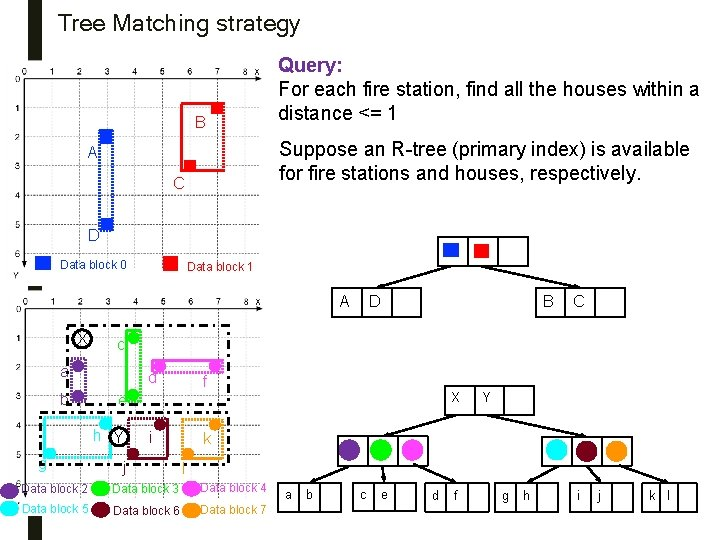Tree Matching strategy B Query: For each fire station, find all the houses within a distance <= 1 Suppose an R-tree (primary index) is available for fire stations and houses, respectively. A C D Data block 0 Data block 1 A X d Data block 2 3 Data block 5 C f X e h Y g B c a b D i j Y k l Data block 3 Data block 4 Data block 6 Data block 7 a b c e d f g h i j k l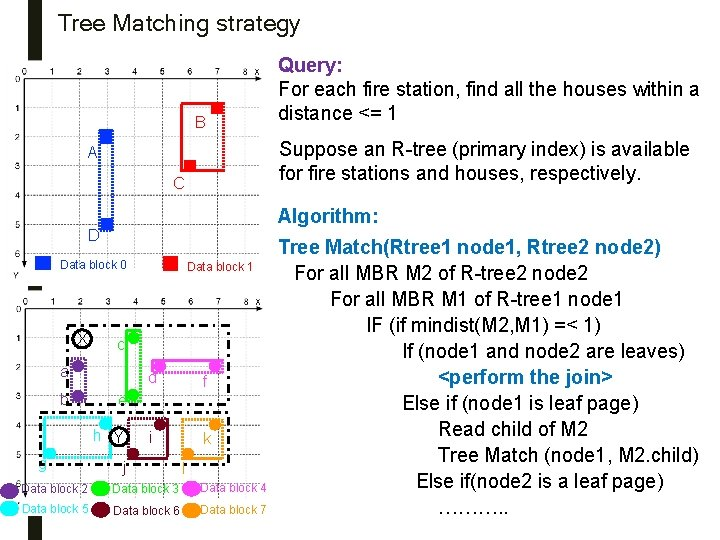Tree Matching strategy B Suppose an R-tree (primary index) is available for fire stations and houses, respectively. A C Algorithm: D Data block 0 X Data block 2 3 Data block 5 d f i k e h Y g Data block 1 c a b Query: For each fire station, find all the houses within a distance <= 1 j l Data block 3 Data block 4 Data block 6 Data block 7 Tree Match(Rtree 1 node 1, Rtree 2 node 2) For all MBR M 2 of R-tree 2 node 2 For all MBR M 1 of R-tree 1 node 1 IF (if mindist(M 2, M 1) =< 1) If (node 1 and node 2 are leaves) <perform the join> Else if (node 1 is leaf page) Read child of M 2 Tree Match (node 1, M 2. child) Else if(node 2 is a leaf page) ………. .Tree Matching strategy B Query: For each fire station, find all the houses within a distance <= 1 Suppose an R-tree (primary index) is available for fire stations and houses, respectively. A C D Data block 0 Data block 1 A X d Data block 2 3 Data block 5 C f X e h Y g B c a b D i j Y k l Data block 3 Data block 4 Data block 6 Data block 7 a b c e d f g h i j k l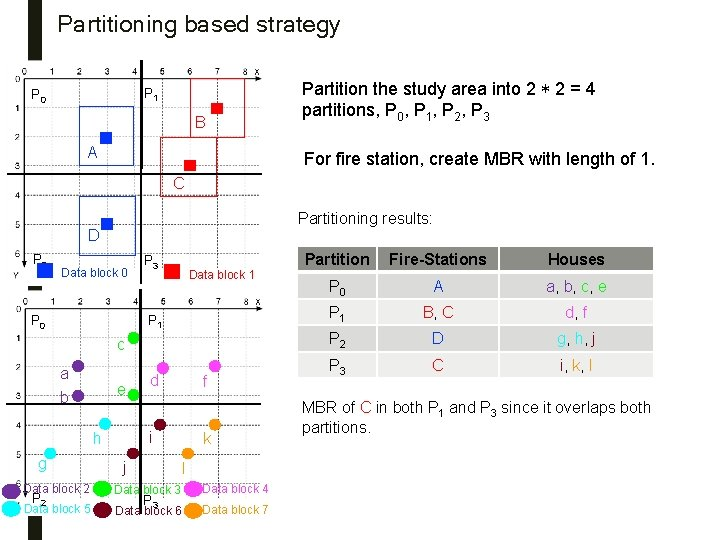Partitioning based strategy P 1 P 0 B A Partition the study area into 2 * 2 = 4 partitions, P 0, P 1, P 2, P 3 For fire station, create MBR with length of 1. C Partitioning results: D P 2 Data block 0 P 3 Data block 1 P 0 c a b e f i h g d j k l Data block 2 Data block 3 Data block 4 Data block 5 Data block 6 Data block 7 P 32 P 3 Partition Fire-Stations Houses P 0 A a, b, c, e P 1 B, C d, f P 2 D g, h, j P 3 C i, k, l MBR of C in both P 1 and P 3 since it overlaps both partitions.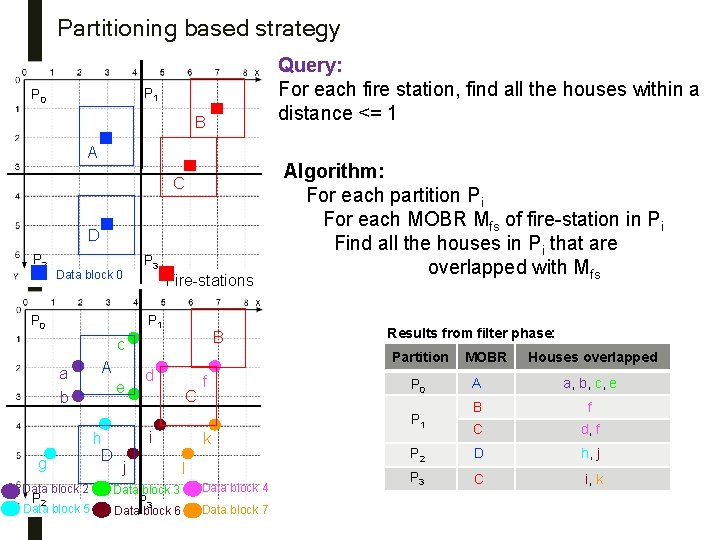Partitioning based strategy Query: For each fire station, find all the houses within a distance <= 1 P 0 B A C D P 2 P 3 Data block 0 Fire-stations P 1 P 0 B c a b g A e h D Results from filter phase: Partition d C i j f k l Data block 2 Data block 3 Data block 4 Data block 5 Data block 6 Data block 7 P 32 Algorithm: For each partition Pi For each MOBR Mfs of fire-station in Pi Find all the houses in Pi that are overlapped with Mfs P 3 MOBR Houses overlapped A a, b, c, e B f C d, f P 2 D h, j P 3 C i, k P 0 P 1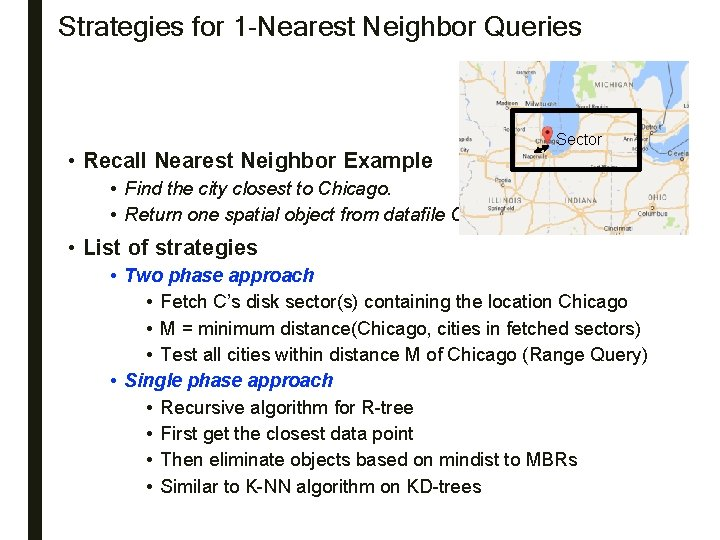Strategies for 1 -Nearest Neighbor Queries • Recall Nearest Neighbor Example Sector • Find the city closest to Chicago. • Return one spatial object from datafile C • List of strategies • Two phase approach • Fetch C’s disk sector(s) containing the location Chicago • M = minimum distance(Chicago, cities in fetched sectors) • Test all cities within distance M of Chicago (Range Query) • Single phase approach • Recursive algorithm for R-tree • First get the closest data point • Then eliminate objects based on mindist to MBRs • Similar to K-NN algorithm on KD-trees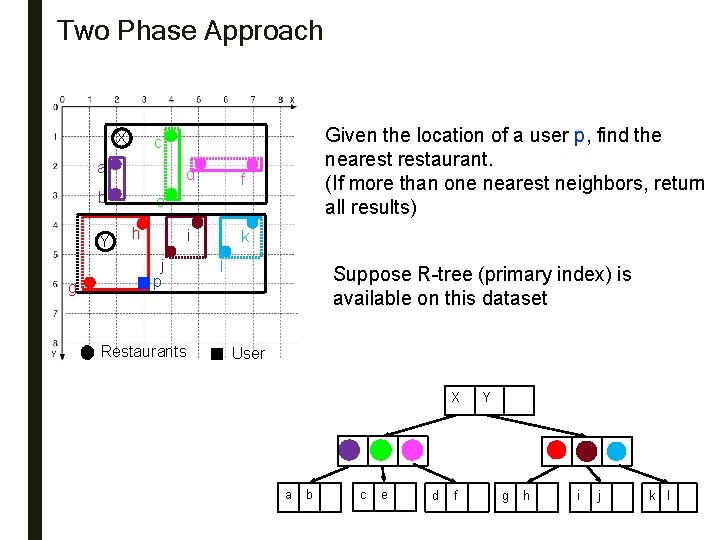Two Phase Approach X a b Y g 3 Given the location of a user p, find the nearestaurant. (If more than one nearest neighbors, return all results) c d f i k e h j p Restaurants l Suppose R-tree (primary index) is available on this dataset User X a b c e d f Y g h i j k l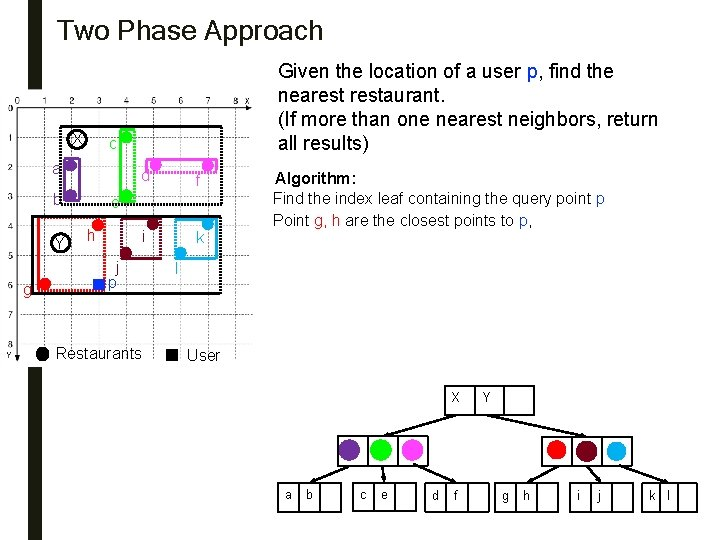Two Phase Approach X c a b Y g 3 Given the location of a user p, find the nearestaurant. (If more than one nearest neighbors, return all results) d f i k e h j p Restaurants Algorithm: Find the index leaf containing the query point p Point g, h are the closest points to p, l User X a b c e d f Y g h i j k lTwo Phase Approach X c a b Y g 3 Given the location of a user p, find the nearestaurant. (If more than one nearest neighbors, return all results) d f i k e h j p Restaurants l User Algorithm: Find the index leaf containing the query point p Point g, h are the closest points to p at distance d. B Create a circle Circlep whose center is p, and radius = d. B Create the MOBR of Circlep : Mp Range query: Mp, and test all points in Mp Root -> Y -> leaves containing <g, h> and <j, i> Since dist(p, j) = 1. 41 < DB, point j is nearest neighbor of p X a b c e d f Y g h i j k lOne Phase Approach – Recursive search on Rtree Given the location of a user p, find the X c a d b Y g 3 nearestaurant. (If more than one nearest neighbors, return all results) f e h First level: i j p Algorithm: k l Second level: Node Min. Dist Max. Dist X 3 7. 47 Nothing X eliminated Y 0 4. 47 Nothing eliminated 3. 16 4. 12 3. 16 5. 10 4. 47 0 2. 83 1. 41 2. 83 3. 16 Node eliminated In the first part of the algorithm we get that Point g, h are the closest points to p, distance = 2